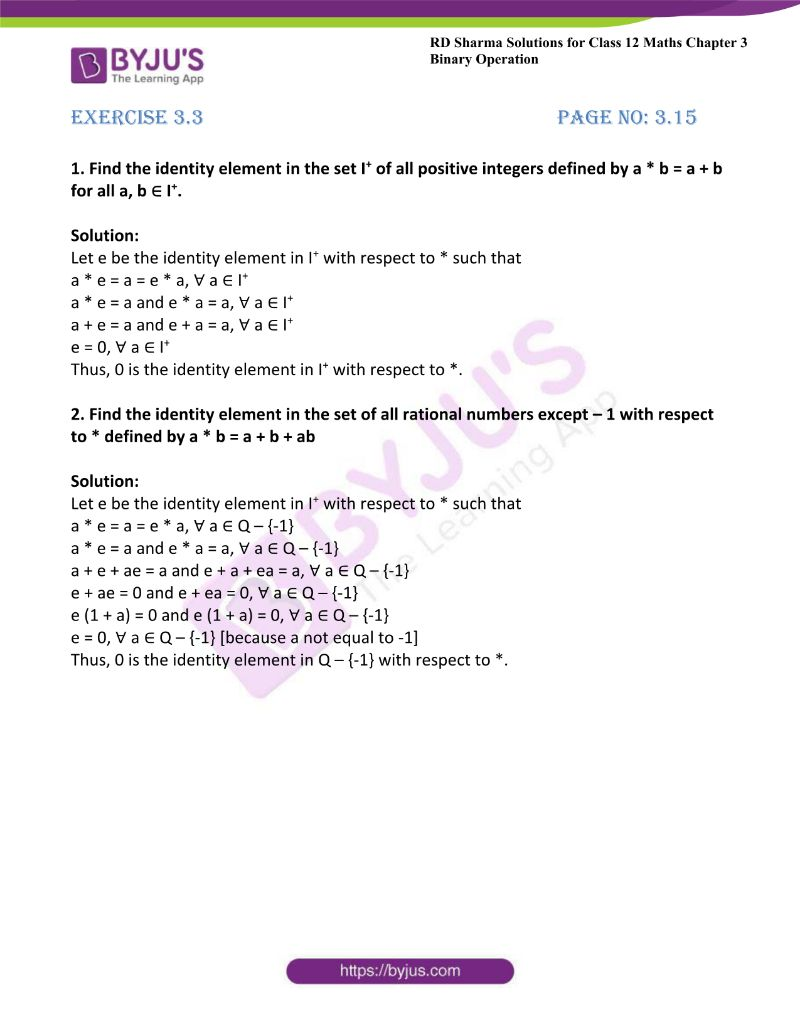# RD Sharma Solutions For Class 12 Maths Exercise 3.3 Chapter 3 Binary Operations

RD Sharma Solutions Class 12 Maths Exercise 3.3 Chapter 3 Binary Operations is provided here. The third exercise of Chapter 3 explains identity elements for the binary operations. The solutions are explained in understandable language which improves grasping abilities among students. The set of experts at BYJU’S conduct vast research on each topic before designing the solutions as per prescribed syllabus of the CBSE board. For more knowledge about the concepts which are covered in this exercise, students can refer to RD Sharma Solutions Class 12 Maths Chapter 3 Binary Operations Exercise 3.3 PDF, which are available here.

## Download the PDF of RD Sharma Solutions For Class 12 Maths Chapter 3 – Binary Operations Exercise 3.3### Access Other Exercises of RD Sharma Solutions For Class 12 Maths Chapter 3 – Binary Operations

Exercise 3.1 Solutions

Exercise 3.2 Solutions

Exercise 3.4 Solutions

Exercise 3.5 Solutions

### Access RD Sharma Solutions For Class 12 Maths Chapter 3 – Binary Operations Exercise 3.3

1. Find the identity element in the set I+ of all positive integers defined by a * b = a + b for all a, b ∈ I+.

Solution:

Let e be the identity element in I+ with respect to * such that

a * e = a = e * a, ∀ a ∈ I+

a * e = a and e * a = a, ∀ a ∈ I+

a + e = a and e + a = a, ∀ a ∈ I+

e = 0, ∀ a ∈ I+

Thus, 0 is the identity element in I+ with respect to *.

2. Find the identity element in the set of all rational numbers except – 1 with respect to * defined by a * b = a + b + ab

Solution:

Let e be the identity element in I+ with respect to * such that

a * e = a = e * a, ∀ a ∈ Q – {-1}

a * e = a and e * a = a, ∀ a ∈ Q – {-1}

a + e + ae = a and e + a + ea = a, ∀ a ∈ Q – {-1}

e + ae = 0 and e + ea = 0, ∀ a ∈ Q – {-1}

e (1 + a) = 0 and e (1 + a) = 0, ∀ a ∈ Q – {-1}

e = 0, ∀ a ∈ Q – {-1} [because a not equal to -1]

Thus, 0 is the identity element in Q – {-1} with respect to *.